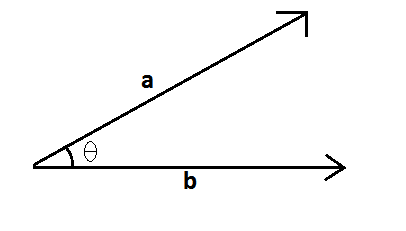# Dot Product Formula

The geometric definition of the dot product states that the dot product between two vectors $\vec{a}$ and $\vec{b}$ is –$\LARGE a.b=\left | a \right |\left | b \right |\cos \theta$

Where θ is the angle between vectors $\vec{a}$ and $\vec{b}$. This formula gives a clear picture on the properties of the dot product. The formula for the dot product in terms of vector components would make it easier to calculate the dot product between two given vectors. The dot product is also known as Scalar product. The symbol for dot product is represented by a heavy dot (.)

Here,

|a| is the magnitude (length) of vector $\vec{a}$
|b| is the magnitude (length) of vector $\vec{b}$
θ is the angle between $\vec{a}$ and $\vec{b}$

#### Dot Product Formula for Two Vectors

$\LARGE a.b=a_{1}a_{2}+b_{1}b_{2}+c_{1}c_{2}$

If we have two vectors a = $a_{1}$, $a_{2}$, $a_{3}$…..$a_{n}$ and b = $b_{1}$, $b_{2}$, $b_{3}$…..$b_{n}$, then the dot product is given by

$\LARGE a.b=a_{1}b_{1}+a_{2}b_{2}+a_{3}b_{3}+…..+a_{n}b_{n}=\sum_{j=1}^{n}a_{j}b_{j}$

### Solved Example

Question: Find the dot product of two vectors $\vec{a}$ and $\vec{b}$ if $\vec{a}$ = 4 $\hat{i}$ + 2 $\hat{j}$ + 1 $\hat{k}$ and $\vec{b}$ = 5 $\hat{i}$ + 4 $\hat{j}$ + $\hat{k}$.
Solution:
Given: $\vec{a}$ = 4 $\hat{i}$ + 2 $\hat{j}$ + 1 $\hat{k}$
$\vec{b}$ = 5 $\hat{i}$ + 4 $\hat{j}$ + $\hat{k}$
The Dot product is given by $\vec{a}$.$\vec{b}$ = a1a2 + b1b2 + c1c2
= 5 $\times$ 4 + 2 $\times$ 4 + 1 $\times$ 1
= 20 + 8 + 1
= 29